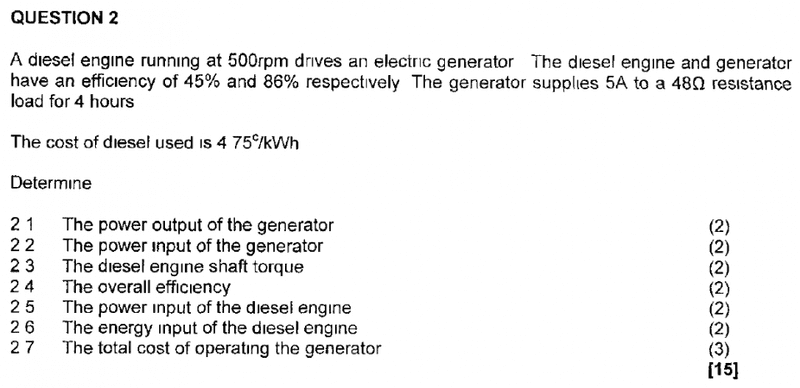# Power output/input, energy input, efficiency of engine & generator

• DevonZA
In summary, the equations indicate that a diesel engine produces 3100.78 watts when running for four hours.

## Homework Statement## Homework Equations

P=2piNT/60
P=I^2R
Pin=Pout/efficieny
E=P/t

## The Attempt at a Solution

2.1 P=I^2R
= (5)^2x48
= 1200W

2.2 Pin=Pout/efficiency
= 1200/0.86
= 1395.35W

2.3 P=2piNT/60
*3100.78=2pi(500)T/60
T=0.016Nm.

2.4 Total efficiency = (45/100x86/100)x100% = 38.7%

2.5 Pin=Pout/efficiency
= 1395.35/0.45
= 3100.78W*

2.6 E=P/t
= 3100.78/4x60x60
= 0.215J

2.7 3.1kWx4hoursxR4.75/kWh = R58.90

I don't feel satisfied that these answers are correct, probably because they are not.
The answer to 2.6 concerns me most.
When can I use the equations P=I^2R and E=I^Rt?

DevonZA said:

## Homework Statement

View attachment 100189

## Homework Equations

P=2piNT/60
P=I^2R
Pin=Pout/efficieny
E=P/t

## The Attempt at a Solution

2.1 P=I^2R
= (5)^2x48
= 1200W

2.2 Pin=Pout/efficiency
= 1200/0.86
= 1395.35W

2.3 P=2piNT/60
*3100.78=2pi(500)T/60
T=0.016Nm.
Extremely small torque output for a diesel engine. You would do much better turning a hand crank.

However, you overlooked the fact that the power output of the diesel engine = power input of the generator.

The efficiency for the diesel engine comes from how much energy in the fuel is converted to useful work at the shaft.
2.4 Total efficiency = (45/100x86/100)x100% = 38.7%

2.5 Pin=Pout/efficiency
= 1395.35/0.45
= 3100.78W*

2.6 E=P/t
= 3100.78/4x60x60
= 0.215J
What are the derived units for watts? Why have you used hours?
2.7 3.1kWx4hoursxR4.75/kWh = R58.90

I don't feel satisfied that these answers are correct, probably because they are not.
The answer to 2.6 concerns me most.
When can I use the equations P=I^2R and E=I^Rt?

SteamKing said:
Extremely small torque output for a diesel engine. You would do much better turning a hand crank.

However, you overlooked the fact that the power output of the diesel engine = power input of the generator.

The efficiency for the diesel engine comes from how much energy in the fuel is converted to useful work at the shaft.

What are the derived units for watts? Why have you used hours?

Hi Steamking

Power input of gen = 1395.35W = power output of engine

P=2pi(N)(T)/60
1395.35=2pi(500)T/60
T=1850.64Nm
That's better :)

The efficiency for the diesel engine comes from how much energy in the fuel is converted to useful work at the shaft.

I'm lost here. Isn't the efficiency the output power/input power x 100%?

Derived unit for watts..power? I have used hours because I need to calculate kw/h?

DevonZA said:
Hi Steamking

Power input of gen = 1395.35W = power output of engine

P=2pi(N)(T)/60
1395.35=2pi(500)T/60
T=1850.64Nm
That's better :)

The efficiency for the diesel engine comes from how much energy in the fuel is converted to useful work at the shaft.

I'm lost here. Isn't the efficiency the output power/input power x 100%?
You can only measure the output power of the diesel engine.

The amount of heat input theoretically furnished by the fuel can be estimated if you know the fuel consumption of the engine, which in this case you don't.However, given the efficiency of the engine, you can work back and estimate how much fuel the engine burns in a given amount of time.
Derived unit for watts..power? I have used hours because I need to calculate kw/h?
Kilowatt-hours is a unit of energy, not kilowatts per hour. The definition of the watt is the same as it always was - the amount of work performed in joules per second.

https://en.wikipedia.org/wiki/Kilowatt_hour

I will look at this again in the morning, it is almost midnight here.